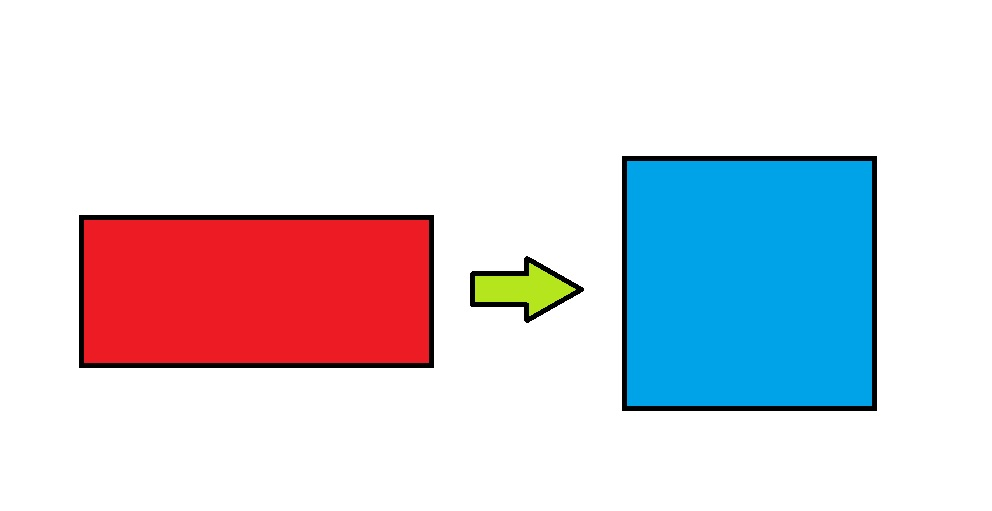# Prime Square

A red rectangle is reshaped into a blue square, as shown below, such that both quadrilaterals have the same perimeter. The area of the square is $4\text{ cm}^2$ larger than that of the rectangle.

If all the sides of both quadrilaterals have prime number lengths $($in $\text{cm}),$ then what is the perimeter of the square?×